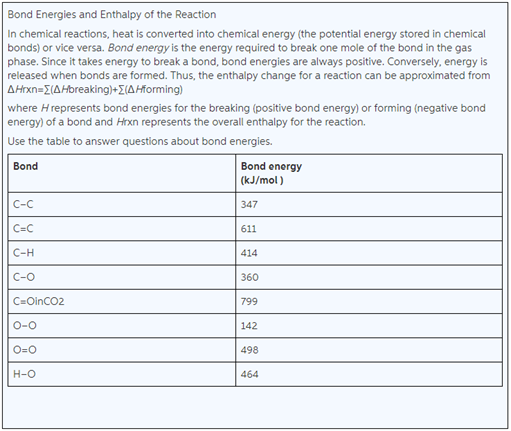Problem: Calculate the bond energy per mole for forming all the bonds of water molecules, H2O. Express your answer to three significant figures and include the appropriate units.

FREE Expert Solution
82% (147 ratings)
FREE Expert Solution
82% (147 ratings)Problem Details

Calculate the bond energy per mole for forming all the bonds of water molecules, H2O. Express your answer to three significant figures and include the appropriate units.What scientific concept do you need to know in order to solve this problem?

Our tutors have indicated that to solve this problem you will need to apply the Bond Energy concept. You can view video lessons to learn Bond Energy Or if you need more Bond Energy practice, you can also practice Bond Energy practice problems .

What is the difficulty of this problem?

Our tutors rated the difficulty of Calculate the bond energy per mole for forming all the bonds... as low difficulty.

How long does this problem take to solve?

Our expert Chemistry tutor, Sabrina took 2 minutes to solve this problem. You can follow their steps in the video explanation above.

What professor is this problem relevant for?

Based on our data, we think this problem is relevant for Professor Hoeger's class at UCSD.